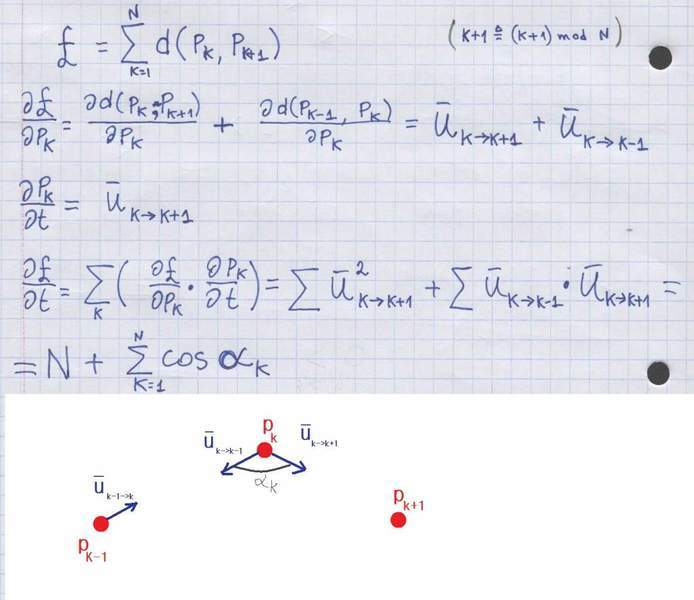# Dog Chase

8daysAweek

## Homework Statement

There are N dogs on the plane. Each dog chases the tail of the next dog with a speed of 1 (and the last dog is chasing the first). I want to prove that the dogs will eventually meet at a single point.
2. The attempt at a solution

I defined a function that is sum of distances between each dog and the next one. If I show that the derivative of this function over time is negative, then this distances will eventually become zero.
I have attached a scan of my attempt. As you can see, the result is not always negative. I know there are better way to solve this problem, I just want to understand what is wrong in my approach. Maybe the derivative at line 2 is wrong?

Thank you.

3. Relevant equations$$p_k$$ is the location of the kth dog on 2D plane.
$$u_k$$ is the unit vector that points to the next dog, it is also the velocity of the kth dog.

#### Attachments

betel
I do not understand where the second equality in your second lines comes from.
You regard your u's as vectors, but treat your p as 1 dimensional point.
It might be a good idea to write the distances explicitly in terms of the x and y coordinates of the positions of the dog. You can then also express the u in terms of the positions.

8daysAweek
p is a 2D point. I forgot to mention that d is the distance function between two points.
I got this result using the chain rule:
$$$\frac{{\partial d\left( {{p_i},{p_j}} \right)}}{{\partial {p_i}}} = \frac{{\partial \sqrt {{{\left( {{p_i} - {p_j}} \right)}^2}} }}{{\partial {p_i}}} = \frac{{\frac{1}{2}}}{{\sqrt {{{\left( {{p_i} - {p_j}} \right)}^2}} }} \cdot 2\left( {\left( {{p_i} - {p_j}} \right)} \right) \cdot 1 = \frac{{{p_i} - {p_j}}}{{d\left( {{p_i} - {p_j}} \right)}} = {u_{i \to j}}$$$

betel
Ok, then your definitions are alright.

I don't have much time right now.
Did you remember that the sum of your angles has to be 2Pi (maybe a multiple of that). Maybe this will help you.

betel
Actually as your result goes, the derivative is always positive.

But you made a small mistake in your second line, that also shows in your last post.

As you defined it, $$\vec u_{k\to k+1}$$ is the vector going from $$p_k$$ to $$p_{k+1}$$, or in vector notation $$\vec u_{k\to k+1}=\vec p_{k+1}-\vec p_k$$.
Therefore
$$$\frac{\partial d\left( p_i,p_j \right)}{\partial p_i} =- u_{i \to j}$$$
This will give you a minus in front of the N in your final result and then the derivative is always negative.